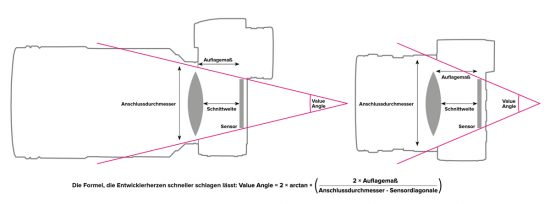# Comparing mirrorless systems based on Fujifilm’s concept of “Value Angle”At the last X Summit Fujifilm introduced the concept of "Value Angle" proposed to measure and compare the potential of a given mirrorless camera system. In general: a bigger/higher Value Angle value (see table above) represents a generally easier way to construct high-quality lenses - see the related portion of Fuji's presentation:

"This number includes parameters Connection diameter, sensor diagonal and flange are summarized. The idea behind this is that the largest possible value angle is generally advantageous and construction of lenses can accommodate that provide a particularly high image quality.

The formula for calculating the value Angle is as follows:
Value Angle = 2 x arctan ((2 × flange) / (connection diameter - Sensor diagonal))

This formula but you do not know and understand, because the value angle can be represented graphically also. When you draw in a cross section through the camera two straight lines each connecting a corner of the sensor and an edge of the lens mount, the angle formed by these lines, the searched value is Angle.

Thus the value Angle takes a very large value, the flange must be as short as possible and - in line with what Olympus had already called for 16 years ago - the connection diameter significantly larger than the sensor diagonal to be.

The mirrorless camera systems, the flange is usually between 16 and 20 mm - the SLR systems have pad dimensions of more than 40 mm. Would still not a mechanical focal plane shutter are placed in front of the sensor, one could reduce the flange back even to 10 to 15 mm. The shortest focal distance, ie the distance of the rear lens to the sensor, can usually be shorter - the lens then protrudes into the camera body, wherein Fuji film X-system, for example by up to 7.5 mm, so that the shortest focal distance is 10.2 mm , In general, the manufacturer does not provide this value, which is unfortunate, because for the interests of the optics, the focal distance is crucial - not the flange (see ProfiFoto 12/2018, page 72)."

Keep in mind that "Value Angle" was developed by Fujifilm. I am sure the companies behind the L-mount alliance will disagree with this concept.

Via Profifoto

•